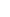30. A SAMPLE MODEL COMPATIBLE WITH (C+V) (C-V) MATHEMATICSNow we can focus on the question why (c+v) (c-v) mathematics exist in nature.I prepared the two figures below to transfer (c+v) (c-v) mathematics to a mechanical setup. Id like to attract your attention to the similarity between these two figures.In the first figure, a signal source (which can also be a regular source of light) sends a signal towards the man. In cases when the cart that the man is on moves forward and backward, the wavelength of the signal that goes to the vehicle changes. The speed of the INCOMING signal relative to the man is always c. The rules of (c+v) (c-v) mathematics as we know. I am not going to repeat these as we have dealt with this topic a lot.In the second figure, the source of the signal is a mechanical arm mounted to the floor. While the arm is swinging up and down at a constant speed, a pen attached to the end of the arm draws on the paper on the cart. The paper goes toward the man at a constant speed (I used c for the speed of the paper to have exact similarity with the figure above.). As a result of these two movements, the drawing that is created is like a regular sine wave. In the cases when the cart moves forward or backward, the wavelength of the drawing changes since the speed of the paper changes relative to the pen. If the cart goes towards the woman, the wavelength shortens; and if it moves away from the woman, the wavelength lengthens. As the speed of the paper is the same as the man on the cart, whether the cart moves or not, the speed of the INCOMING signal relative to the man doesnt change. The case is different for the woman, though; the movement of the cart changes the speed of the paper relative to the woman and, as a result of this, the speeds of the waves change relative to the woman. If the cart moves away from the woman, the OUTGOING waves that go towards the man will speed up and they will slow down if the cart approaches the woman. In this way, we obtained a model fully compatible with (c+v) (c-v) mathematics.We need to think now. (c+v) (c-v) mathematics is valid for both situations described in the figures above. What is the most obvious difference between these two figures? If you look carefully, there is no PAPER in the first figure. In the second figure, however, the waves travel on a PAPER; the PAPER carries the waves. No matter how, nature needs to have a similar foundation to be able to create (c+v) (c-v) mathematics. If we can delineate and describe a PAPER for the first figure based on the similarity between two figures, we can reveal the physical foundation that leads to (c+v) (c-v) mathematics in nature.There are some mathematical equations we found thanks to our studies on gravitational force, electromagnetic force and charge forces and we are using them. However, we dont know much about the mechanism of nature that leads to or carry these forces. Right now, (c+v) (c-v) mathematics made us go a full circle; I mean, even if we dont know the reason of (c+v) (c-v) mathematics, we can reach accurate results by using it in our studies now that we reached mathematical equations and that we understand its rules. This is the practical part of the work. When it comes to the theoretical part of the work, there are some serious questions that need to be answered. I wrote some major questions about (c+v) (c-v) mathematics in the previous chapter.(c+v) (c-v) mathematics gives opportunities and new beginnings in order to discover the mysterious structure of nature, at least related to its own features. Doing experiments and testing ideas regarding (c+v) (c-v) mathematics by using electromagnetic waves are possible, and this is probably the easiest method available to discover the functioning mechanism of nature. Gravitational force, charge forces or other forces cannot provide us with the widespread opportunities to use that electromagnetic waves do. We will undoubtedly make progress on the topic of how forces such as gravitational force, charge forces, and electromagnetic force to the extent that we comprehend the foundation of (c+v) (c-v) mathematics in nature.After this stage, we need to focus our attention on nature. We now know what to look for; we will try to find traces, clues of a very special structure that can take upon the function of PAPER in nature.